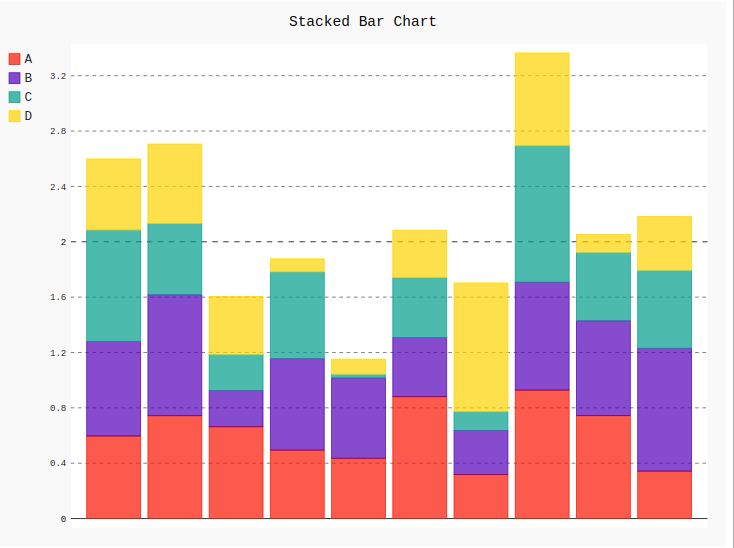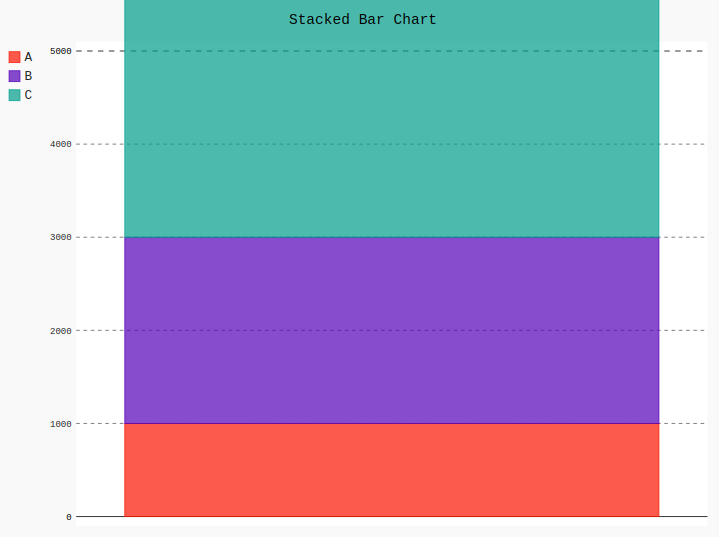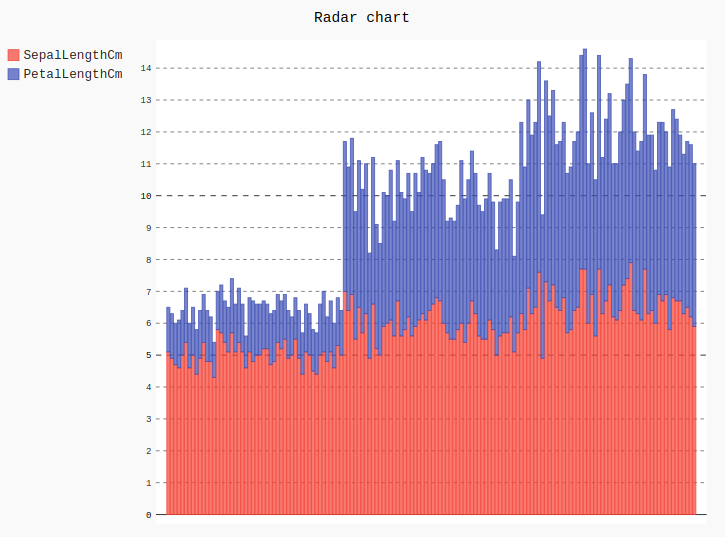# Stacked Bar chart in pygal

• Last Updated : 10 Jul, 2020

Pygal is a Python module that is mainly used to build SVG (Scalar Vector Graphics) graphs and charts. SVG is a vector-based graphics in the XML format that can be edited in any editor. Pygal can create graphs with minimal lines of code that can be easy to understand and write.

## Stacked Bar Chart

This graph is similar to the bar chart but the only difference is that the values are stacked in it. It can be created using the StackedBar() method.

Attention geek! Strengthen your foundations with the Python Programming Foundation Course and learn the basics.

To begin with, your interview preparations Enhance your Data Structures concepts with the Python DS Course. And to begin with your Machine Learning Journey, join the Machine Learning - Basic Level Course

Syntax:

```line_chart = pygal.StackedBar()
```

Example 1:

## Python3

 `# importing pygal``import` `pygal``import` `numpy`` ` ` ` `# creating the chart object``bar_chart ``=` `pygal.StackedBar()`` ` `# naming the title``bar_chart.title ``=` `'Stacked Bar Chart'`        ` ` `# Random data``bar_chart.add(``'A'``, numpy.random.rand(``10``))``bar_chart.add(``'B'``, numpy.random.rand(``10``))``bar_chart.add(``'C'``, numpy.random.rand(``10``))``bar_chart.add(``'D'``, numpy.random.rand(``10``))`` ` `bar_chart`

Output:Example 2:

## Python3

 `# importing pygal``import` `pygal`` ` `# creating the chart object``bar_chart ``=` `pygal.StackedBar()`` ` `# naming the title``bar_chart.title ``=` `'Stacked Bar Chart'`     ` ` `bar_chart.``range` `=` `[``0``, ``5000``]`` ` `# Random data``bar_chart.add(``'A'``, ``1000``)``bar_chart.add(``'B'``, ``2000``)``bar_chart.add(``'C'``, ``3500``)`` ` `bar_chart`

Output:Example 3: Using Iris dataset

## Python3

 `# importing pygal``import` `pygal``import` `pandas`` ` ` ` `# creating the chart object``bar_chart ``=` `pygal.StackedBar()`` ` `# naming the title``bar_chart.title ``=` `'Stacked Bar chart'`` ` `df ``=` `pandas.read_csv(``'Iris.csv'``)`` ` `bar_chart.add(``"SepalLengthCm"``, df[``'SepalLengthCm'``])``bar_chart.add(``"PetalLengthCm"``, df[``'PetalLengthCm'``])``bar_chart`

Output:My Personal Notes arrow_drop_up